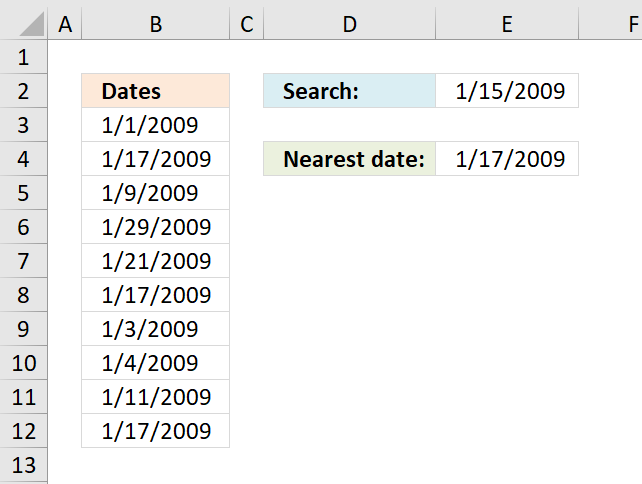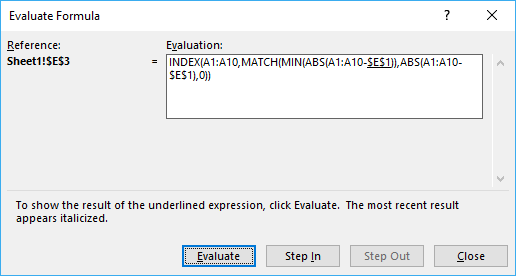Author: Oscar Cronquist Article last updated on July 08, 2018The image above demonstrates an array formula in cell E4 that searches for the closest date in column A to the date in cell E2.

Array formula in E4:

=INDEX(B3:B12, MATCH(MIN(ABS(B3:B12-\$E\$2)), ABS(B3:B12-\$E\$2), 0))

Recommended articles:

Find date range

Elizabeth asks: I need to figure out the date range of a cell. So if cell "E2" has a date […]

Formula for matching a date within a date range

Table of contents Match a date when a date range is entered in a single cell Match a date when […]

### How to enter an array formula

1. Select cell E4
2. Type or copy/paste above array formula to formula bar3. Press and hold Ctrl + Shift
4. Press Enter

Learn more about array formulas:

How to enter an array formula

Array formulas allows you to do advanced calculations not possible with regular formulas.

### Explaining array formula in cell E4

You can easily follow along, select cell E3. Go to tab "Formulas" and click on "Evaluate formula".Click "Evaluate" button to move to next step.

#### Step 1 - Subtract dates with search date

B3:B12-\$E\$2

becomes

{39814; 39830; 39822; 39842; 39834; 39830; 39816; 39817; 39824; 39830}-39828

and returns {-14;2;-6;14;6;2;-12;-11;-4;2}

#### Step 2 - Convert numerical values to absolute values

The ABS function converts a negative number to a positive.

ABS(B3:B12-\$E\$2)

becomes

ABS({-14;2;-6;14;6;2;-12;-11;-4;2})

and returns {14;2;6;14;6;2;12;11;4;2}

#### Step 3 - Find smallest numerical value in array

The MIN function returns the smallest number in a cell range or array.

MIN(ABS(B3:B12-\$E\$2))

becomes

MIN({14;2;6;14;6;2;12;11;4;2})

and returns 2.

#### Step 4 - Find position in array

The MATCH function returns the relative position of a given value in an array or cell range.

MATCH(MIN(ABS(B3:B12-\$E\$2)), ABS(B3:B12-\$E\$2), 0)

becomes

MATCH(2, ABS(B3:B12-\$E\$2), 0)

becomes

MATCH(2, {14;2;6;14;6;2;12;11;4;2}, 0)

and returns 2. Numerical value 2 has position 2 in the array.

Learn more about the MATCH function:

How to use the MATCH function

Identify the position of a value in an array.

#### Step 5 - Return value

INDEX(B3:B12, MATCH(MIN(ABS(B3:B12-\$E\$2)), ABS(B3:B12-\$E\$2), 0))

becomes

INDEX(B3:B12, 2)

becomes

INDEX({39814;39830;39822; 39842;39834;39830; 39816;39817;39824;39830}, 2)

an returns 39830 or 1-17-2009 in cell E4.

Learn more about the INDEX function:

How to use the INDEX function

Gets a value in a specific cell range based on a row and column number.

### Download excel example file

find-nearest-date.xls
(Excel 97-2003 Workbook *.xls)

Recommended articles:

Lookup min max values within a date range

This article explains how to find the smallest and largest value using two conditions. In this case they are date […]

Find latest date based on a condition

Table of contents Lookup a value and find max date How to enter an array formula Explaining array formula Download […]